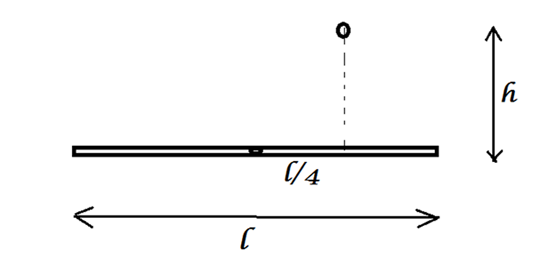# Genius Insect!A Thin rod of length $l$ is hinged at the center. An insect falls on it from a height $h$. The distance of the impact point is $l/4$ from the center.
Apparently, the insect is a Genius. To avoid much dizziness, it devices a method to make the rod rotate at constant angular velocity. The insect starts crawling towards the outer edge of the rod.

Find the height $h$ from which the insect drops, such that by the time the rod becomes vertical, the insect reaches the outer edge.

Details and Assumptions:

• $l = 5m$
• $g=9.8m/s^2$
• The mass of the insect is equal to the mass of the rod
• Neglect air resistance and friction due to the rod.
×

Problem Loading...

Note Loading...

Set Loading...